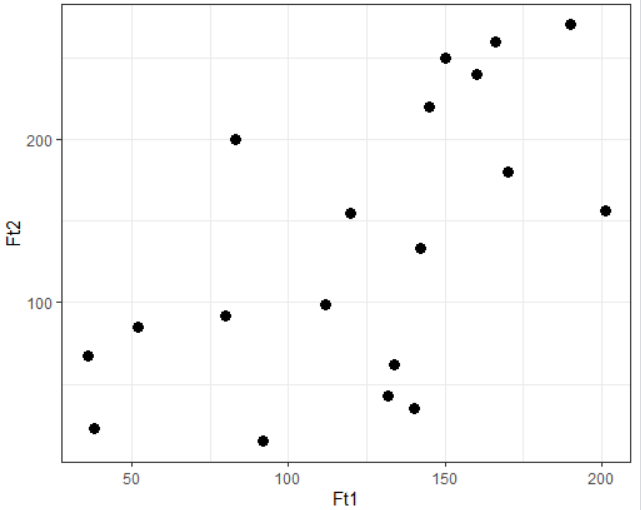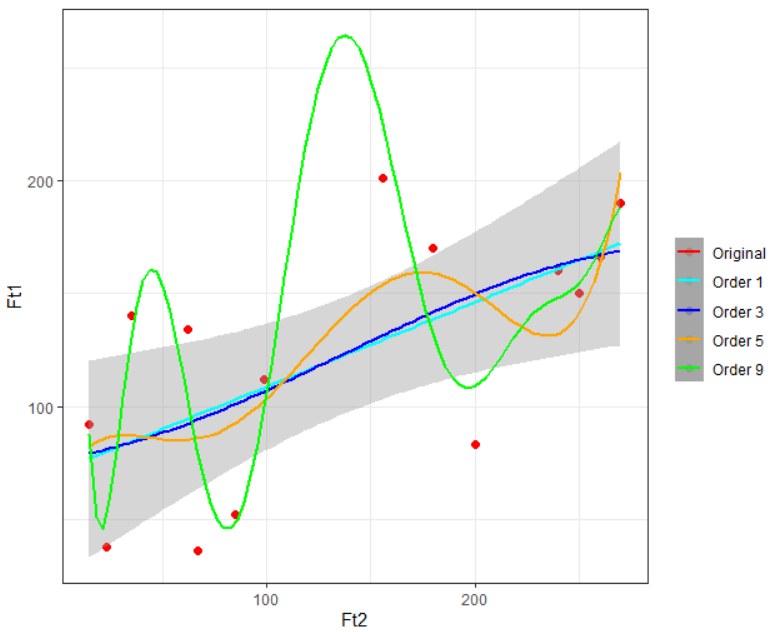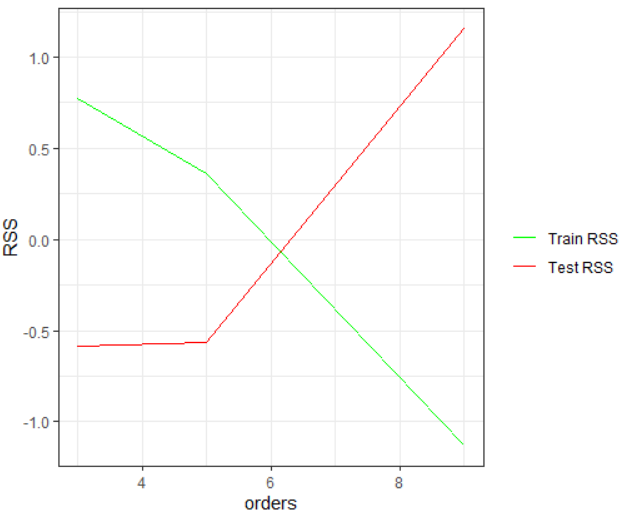Chhaya Wagmi

# Polynomial Functions Analysis with R

• Aug 24, 2020
• 5,761 Views
• Aug 24, 2020
• 5,761 Views
Data Analytics
R
Data
Machine Learning

## Introduction

When you have feature points aligned in almost a straight line, you can use simple linear regression or multiple linear regression (in the case of multiple feature points). But how will you fit a function on a feature(s) whose points are non-linear? In this guide you will learn to implement polynomial functions that are suitable for non-linear points. You will be working in R and should already have a basic knowledge on regression to follow along.

## Describing the Original Data and Creating Train and Test Data

### Original Data

Consider a dependent variable Ft1 and an independent variable Ft2 with 19 data points as shown:

Ft1Ft2
3823
5285
3667
9215
83200
170180
14035
201156
11299
13243
8092
13462
150250
160240
190270
145220
166260
120155
142133

You can visualize the complete data using the `ggplot2` library as shown:

``````1# Load ggplot2 library
2library(ggplot2)
3
4# Load data from a CSV file
6
7# Visualize the data
8ggplot(data) +
9  geom_point(aes(Ft1, Ft2),size=3) +
10  theme_bw()``````
r### Creating Train and Test Data

You can split the original data into train and test in a ratio of 75:25 with the following code:

``````1# Set a seed value for reproducible results
2set.seed(70)
3
4# Split the data
5ind <- sample(x = nrow(data), size = floor(0.75 * nrow(data)))
6
7# Store the value in train and test dataframes
8train <- data[ind,]
9test <- data[-ind,]``````
r

## Building Polynomial Regression of Different Degrees

To build a polynomial regression in R, start with the `lm` function and adjust the `formula` parameter value. You must know that the "degree" of a polynomial function must be less than the number of unique points.

At this point, you have only 14 data points in the `train` dataframe, therefore the maximum polynomial degree that you can have is 13. The given code builds four polynomial functions of degree 1, 3, 5, and 9.

``````1# Order 1
2poly_reg1 <- lm(formula = Ft1~poly(Ft2,1),
3                data = train)
4# Order 3
5poly_reg3 <- lm(formula = Ft1~poly(Ft2,3),
6                data = train)
7# Order 5
8poly_reg5 <- lm(formula = Ft1~poly(Ft2,5),
9                data = train)
10# Order 9
11poly_reg9 <- lm(formula = Ft1~poly(Ft2,9),
12                data = train)``````
r

Once you have successfully built these four models you can visualize them on your training data using the given `ggplot` code:

``````1ggplot(train) +
2  geom_point(aes(Ft2, Ft1, col = "Original"), cex = 2) +
3  stat_smooth(method = "lm", formula = y~poly(x,1), aes(Ft2, poly_reg1\$fitted.values, col = "Order 1")) +
4  stat_smooth(method = "lm", formula = y~poly(x,3), aes(Ft2, poly_reg3\$fitted.values, col = "Order 3")) +
5  stat_smooth(method = "lm", formula = y~poly(x,5), aes(Ft2, poly_reg5\$fitted.values, col = "Order 5")) +
6  stat_smooth(method = "lm", formula = y~poly(x,9), aes(Ft2, poly_reg9\$fitted.values, col = "Order 9")) +
7  scale_colour_manual("",
8                      breaks = c("Original",  "Order 1", "Order 3", "Order 5", "Order 9"),
9                      values = c("red","cyan", "blue","orange","green")) +
10  theme_bw()``````
r## Measuring the RSS Value on Train and Test Data

You have all the information to get the RSS value on train data, but to get the RSS value of test data, you need to predict the Ft1 values. Use the given code to do so:

``````1# Predicting values using test data by each model
2poly1_pred <- predict(object = poly_reg1,
3                      newdata =  data.frame(Ft2 = test\$Ft2))
4poly3_pred <- predict(object = poly_reg3,
5                      newdata =  data.frame(Ft2 = test\$Ft2))
6poly5_pred <- predict(object = poly_reg5,
7                      newdata =  data.frame(Ft2 = test\$Ft2))
8poly9_pred <- predict(object = poly_reg9,
9                      newdata =  data.frame(Ft2 = test\$Ft2))``````
r

Now, you can find RSS values for both the data as shown:

``````1# RSS for train data based on each model
2train_rss1 <- mean((train\$Ft1 - poly_reg1\$fitted.values)^2)  # Order 1
3train_rss3 <- mean((train\$Ft1 - poly_reg3\$fitted.values)^2)  # Order 3
4train_rss5 <- mean((train\$Ft1 - poly_reg5\$fitted.values)^2)  # Order 5
5train_rss9 <- mean((train\$Ft1 - poly_reg9\$fitted.values)^2)  # Order 9
6
7# RSS for test data based on each model
8test_rss1 <- mean((test\$Ft1 - poly1_pred)^2)  # Order 1
9test_rss3 <- mean((test\$Ft1 - poly3_pred)^2)  # Order 3
10test_rss5 <- mean((test\$Ft1 - poly5_pred)^2)  # Order 5
11test_rss9 <- mean((test\$Ft1 - poly9_pred)^2)  # Order 9``````
r
0.0001673.8671405.703436.5995
42004.6972669.9034725.91725385.6354

From the above two tables you can observe that the RSS value for train data starts to decrease after the first degree, which means the higher the degree better the curve fitting and reduced error. However, at the same time the test RSS increases with the increase of the degree, which implies underfitting. You can observe these patterns from the given plot.

``````1# Visualizing train and test RSS for each model
2
3# excluding degree 1
6orders <- c(3, 5, 9)
7
8ggplot() +